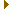# mightymandel v16

## GPU-based Mandelbrot set explorer

atom.h File Reference

### (v16)

Mandelbrot set atom finding. More...

#include <mpfr.h>
#include "utility.h"Include dependency graph for atom.h:This graph shows which files directly or indirectly include this file:

Go to the source code of this file.

## Functions

int crosses_positive_real_axis (const mpfr_t ax, const mpfr_t ay, const mpfr_t bx, const mpfr_t by)
Check if a line segment intersects with the positive real axis.
int surrounds_origin (const mpfr_t ax, const mpfr_t ay, const mpfr_t bx, const mpfr_t by, const mpfr_t cx, const mpfr_t cy, const mpfr_t dx, const mpfr_t dy)
Check if a square surrounds the origin.
int did_escaped (const mpfr_t x, const mpfr_t y)
Check if a point escaped.
unsigned int boxperiod (const mpfr_t cx, const mpfr_t cy, const mpfr_t r, unsigned int maxperiod, void *abort_data, abort_t abort_fn)
Find the lowest period of any atom inside a box.
int muatom (int period, mpfr_t x, mpfr_t y, mpfr_t z, void *abort_data, abort_t abort_fn)
Find an atom of a given period.

## Detailed Description

Mandelbrot set atom finding.

Definition in file atom.h.

## Function Documentation

 unsigned int boxperiod ( const mpfr_t cx, const mpfr_t cy, const mpfr_t r, unsigned int maxperiod, void * abort_data, abort_t abort_fn )

Find the lowest period of any atom inside a box.

Uses the Jordan curve method described here: http://mrob.com/pub/muency/period.html.

Parameters
 cx The real part of the box center. cy The imaginary part of the box center. r The box radius. maxperiod The maximum period to check until.
Returns
0 if no period was found, otherwise the found period.

Definition at line 87 of file atom.c.

References did_escaped(), and surrounds_origin().

Referenced by fpxx_start().Here is the call graph for this function:Here is the caller graph for this function:

 int crosses_positive_real_axis ( const mpfr_t ax, const mpfr_t ay, const mpfr_t bx, const mpfr_t by )

Check if a line segment intersects with the positive real axis.

Parameters
 ax The x coordinate of the first point. ay The y coordinate of the first point. bx The x coordinate of the second point. by The y coordinate of the second point.
Returns
1 if $$(a,b)$$ intersects $$(0,+\infty)$$, 0 otherwise.

Definition at line 23 of file atom.c.

Referenced by surrounds_origin().Here is the caller graph for this function:

 int did_escaped ( const mpfr_t x, const mpfr_t y )

Check if a point escaped.

This is a cheap inaccurate check using exponents. Inf/NaN are assumed escaped.

Parameters
 x The real part of the point. y The imaginary part of the point.
Returns
1 if the point escaped, 0 otherwise.

Definition at line 71 of file atom.c.

Referenced by boxperiod().Here is the caller graph for this function:

 int muatom ( int period, mpfr_t x, mpfr_t y, mpfr_t z, void * abort_data, abort_t abort_fn )

Find an atom of a given period.

Uses Newton's method as described here: http://mathr.co.uk/mandelbrot/bonds.pdf. The method is iterated with increased precision until the nucleus and $$\frac{1}{2}$$ bond are distint, which gives a size estimate and ensures the nucleus has sufficient precision.

Parameters
 period The period of the nucleus. x The real part of the nucleus (input: guess, output: nucleus). y The imaginary part of the nucleus (input: guess, output: nucleus). z The size of the nucleus (output valid when 1 is returned)
Returns
1 if the nucleus was accurately determined, 0 otherwise.

Definition at line 151 of file atom.c.

References VARS.

Referenced by fpxx_start().Here is the caller graph for this function:

 int surrounds_origin ( const mpfr_t ax, const mpfr_t ay, const mpfr_t bx, const mpfr_t by, const mpfr_t cx, const mpfr_t cy, const mpfr_t dx, const mpfr_t dy )

Check if a square surrounds the origin.

Parameters
 ax The x coordinate of the first point. ay The y coordinate of the first point. bx The x coordinate of the second point. by The y coordinate of the second point. cx The x coordinate of the third point. cy The y coordinate of the third point. dx The x coordinate of the fourth point. dy The y coordinate of the fourth point.
Returns
1 if $$(a,b)(b,c)(c,d)(d,a)$$ surround $$0$$, 0 otherwise.

Definition at line 54 of file atom.c.

References crosses_positive_real_axis().

Referenced by boxperiod().Here is the call graph for this function:Here is the caller graph for this function: